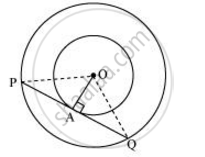Share

# Two concentric circles are of radii 5 cm and 3 cm. Find the length of the chord of the larger circle which touches the smaller circle - CBSE Class 10 - Mathematics

ConceptNumber of Tangents from a Point on a Circle

#### Question

Two concentric circles are of radii 5 cm and 3 cm. Find the length of the chord of the larger circle which touches the smaller circle

#### SolutionLet the two concentric circles be centered at point O. And let PQ be the chord of the larger circle which touches the smaller circle at point A. Therefore, PQ is tangent to the smaller circle.

OA ⊥ PQ (As OA is the radius of the circle)

Applying Pythagoras theorem in ΔOAP, we obtain

OA+ AP= OP2

3+ AP= 52

9 + AP= 25

AP= 16

AP = 4

In ΔOPQ,

Since OA ⊥ PQ,

AP = AQ (Perpendicular from the center of the circle bisects the chord)

∴ PQ = 2AP = 2 × 4 = 8

Therefore, the length of the chord of the larger circle is 8 cm.

Is there an error in this question or solution?

#### APPEARS IN

NCERT Solution for Mathematics Textbook for Class 10 (2019 to Current)
Chapter 10: Circles
Ex. 10.20 | Q: 7 | Page no. 214

#### Video TutorialsVIEW ALL 

Solution Two concentric circles are of radii 5 cm and 3 cm. Find the length of the chord of the larger circle which touches the smaller circle Concept: Number of Tangents from a Point on a Circle.
S